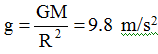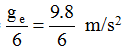Ex﻿

# 4. Gravitation

#### Earth's Gravitational Force

The force which earth exerts on a body is called 'force of gravity'. i.e.Where M = mass of the earth, R = radius of the earth.
Due to this force, a body released from some height on the earth's surface falls towards the earth with its velocity increasing at a constant rate.
Acceleration due to Gravity :
The acceleration produced in a body due to attraction of earth is called the acceleration due to gravity and is denoted by 'g'.near the earth surface g on moon ≈A body moving upwards with some initial velocity, experiences a retardation of 9.8 m/s2 & its velocity decreases continuously unless it becomes zero.
After this, it again starts falling towards the earth with the same acceleration of 9.8 m/s2.
The value of g is minimum at equator and maximum at poles.
The value of g does not depend upon the mass of the body falling towards the earth.

If you want to give information about online courses to other students, then share it with more and more on Facebook, Twitter, Google Plus. The more the shares will be, the more students will benefit. The share buttons are given below for your convenience.
×

#### NTSE Physics (Class X)

• Electricity
• Magnetic Effect of Curent
• Light Reflection
• Light Refraction
• Human Eye & Colourful World
• Source of Energy
• Motion

#### NTSE Physics (Class IX)

• Force & laws of motion
• Work and Energy
• Gravitation
• Sound

SHOW CHAPTERS